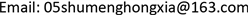﻿ 图的符号全控制数 Signed Total Domination Number of Graphs

Vol. 07  No. 12 ( 2018 ), Article ID: 27994 , 6 pages
10.12677/AAM.2018.712180

Signed Total Domination Number of Graphs

Xia Hong, Feng Gao, Caihuan Zhang, Chunyan Wei

Department of Mathematics, Luoyang Normal University, Luoyang HenanReceived: Nov. 16th, 2018; accepted: Dec. 6th, 2018; published: Dec. 13th, 2018ABSTRACT

Let $G=\left(V,E\right)$ be a graph and denotes $f\left(S\right)={\sum }_{v\in S}f\left(v\right)$ for $S\subseteq V$ . A function $f:V\to \left\{-1,+1\right\}$ is said to be a signed total domination function (STDF), if $f\left(N\left(v\right)\right)\ge 1$ for $v\in V$ . The signed total domination number is ${\gamma }_{st}\left(G\right)=min\left\{f\left(V\right)|f\text{}isanSTDFof\text{}G\right\}$ . In this paper, a lower bound of the signed total domination number are obtained and we determine a exact value of signed total domination number of two classes graphs generalized Petesen graph $P\left(n,k\right)$ and Double generalized Petesen graph $DP\left(n,k\right)$ by exhaustived method and classified discussion, where $n\equiv 0\left(mod\text{\hspace{0.17em}}3\right),$ $k\ne 0\left(mod\text{\hspace{0.17em}}3\right)$ .

Keywords:Signed Total Domination Function, Signed Total Domination Number, Generalized Petesen Graph $P\left(n,k\right)$ , Double Generalized Petesen Graph $DP\left(n,k\right)$1. 引言

2. 基本概念

i) $|{V}_{-1}|+|{V}_{+1}|=n$

ii) ${\gamma }_{st}\left(G\right)=|{V}_{+1}|-|{V}_{-1}|$

$\begin{array}{l}V\left(P\left(n,k\right)\right)=\left\{{u}_{1},{u}_{2},\cdots ,{u}_{n},{v}_{1},{v}_{2},\cdots ,{v}_{n}\right\}\\ E\left(P\left(n,k\right)\right)=\left\{{u}_{i}{u}_{i+1},{u}_{i}{v}_{i},{v}_{i}{v}_{i+k}|i\in {Ζ}_{n}\right\}\end{array}$

$\begin{array}{l}V\left(P\left(n,k\right)\right)=\left\{{x}_{i},{u}_{i},{v}_{i},{y}_{i}|i\in {Ζ}_{n}\right\}\\ E\left(P\left(n,k\right)\right)=\left\{{x}_{i}{x}_{i+1},{y}_{i}{y}_{i+1},{x}_{i}{u}_{i},{y}_{i}{v}_{i},{u}_{i}{v}_{i+k},{v}_{i}{u}_{i+k}|i\in {Ζ}_{n}\right\}\end{array}$

3. 主要定理及其证明

i) $\left(\Delta -1\right)|{V}_{+1}|\ge \left(\delta +1\right)|{V}_{-1}|$

ii) $|{V}_{+1}|\ge \frac{\delta +1}{\Delta +\delta }n$

iii) ${\gamma }_{st}\left(G\right)\ge \frac{\delta +2-\Delta }{\Delta +\delta }n$

$\begin{array}{c}|{V}_{-1}|+|{V}_{+1}|=n\le {\sum }_{v\in V\left(G\right)}f\left(N\left(v\right)\right)={\sum }_{v\in V\left(G\right)}d\left(v\right)f\left(v\right)\\ ={\sum }_{v\in {V}_{+1}}d\left(v\right)-{\sum }_{v\in {V}_{-1}}d\left(v\right)\le \Delta |{V}_{+1}|-\delta |{V}_{-1}|\end{array}$

$\left(\Delta -1\right)|{V}_{+1}|\ge \left(\delta +1\right)|{V}_{-1}|$

ii) 由性质和i)，推导出

$\left(\Delta -1\right)|{V}_{+1}|\ge \left(\delta +1\right)\left(n-|{V}_{+1}|\right)=\delta n+n-\delta |{V}_{+1}|-|{V}_{+1}|$

$|{V}_{+1}|\ge \frac{\delta +1}{\Delta +\delta }n$

iii) 由性质和i)，有

$\left(\Delta +\delta \right){\gamma }_{st}\left(G\right)=\left(\Delta +\delta \right)\left(2|{V}_{+1}|-n\right)\ge 2\left(\delta +1\right)n-\left(\Delta +\delta \right)n=\left(\delta +2-\Delta \right)n$

${\gamma }_{st}\left(G\right)\ge \frac{\delta +2-\Delta }{\Delta +\delta }n$

${\gamma }_{st}\left(P\left(n,k\right)\right)=\frac{2n}{3}$

$\begin{array}{l}V\left(P\left(n,k\right)\right)=\left\{{u}_{1},{u}_{2},\cdots ,{u}_{n},{v}_{1},{v}_{2},\cdots ,{v}_{n}\right\}\\ E\left(P\left(n,k\right)\right)=\left\{{u}_{i}{u}_{i+1},{u}_{i}{v}_{i},{v}_{i}{v}_{i+k}|i\in {Ζ}_{n}\right\}\end{array}$

$|V\left(P\left(n,k\right)\right)|=2n,\text{\hspace{0.17em}}\text{\hspace{0.17em}}|E\left(P\left(n,k\right)\right)|=3n$

${\gamma }_{st}\left(P\left(n,k\right)\right)\ge \frac{2n}{3}$

$f\left({u}_{i}\right)=\left\{\begin{array}{l}+1,当i\equiv 0,2\left(\mathrm{mod}3\right)\\ -1,当i\equiv 1\left(\mathrm{mod}3\right)\end{array}$

$f\left({v}_{i}\right)=\left\{\begin{array}{l}+1,当i\equiv 0,2\left(\mathrm{mod}3\right)\\ -1,当i\equiv 1\left(\mathrm{mod}3\right)\end{array}$

$f\left(V\left(G\right)\right)=s-t=\frac{2n}{3}$

${\gamma }_{st}\left(P\left(n,k\right)\right)\le \frac{2n}{3}$

${\gamma }_{st}\left(DP\left(n,k\right)\right)=\frac{4n}{3}$

$\begin{array}{l}V\left(P\left(n,k\right)\right)=\left\{{x}_{i},{u}_{i},{v}_{i},{y}_{i}|i\in {Ζ}_{n}\right\}\\ E\left(P\left(n,k\right)\right)=\left\{{x}_{i}{x}_{i+1},{y}_{i}{y}_{i+1},{x}_{i}{u}_{i},{y}_{i}{v}_{i},{u}_{i}{v}_{i+k},{v}_{i}{u}_{i+k}|i\in {Ζ}_{n}\right\}\end{array}$

$|V\left(P\left(n,k\right)\right)|=4n,\text{\hspace{0.17em}}\text{\hspace{0.17em}}|E\left(P\left(n,k\right)\right)|=5n$

${\gamma }_{st}\left(P\left(n,k\right)\right)\ge \frac{4n}{3}$

$f\left({x}_{i}\right)=\left\{\begin{array}{l}+1,当i\equiv 0,2\left(\mathrm{mod}3\right)\\ -1,当i\equiv 1\left(\mathrm{mod}3\right)\end{array}$

$f\left({u}_{i}\right)=\left\{\begin{array}{l}+1,当i\equiv 0,2\left(\mathrm{mod}3\right)\\ -1,当i\equiv 1\left(\mathrm{mod}3\right)\end{array}$

$f\left({v}_{i}\right)=\left\{\begin{array}{l}+1,当i\equiv 0,2\left(\mathrm{mod}3\right)\\ -1,当i\equiv 1\left(\mathrm{mod}3\right)\end{array}$

$f\left({y}_{i}\right)=\left\{\begin{array}{l}+1,当i\equiv 0,2\left(\mathrm{mod}3\right)\\ -1,当i\equiv 1\left(\mathrm{mod}3\right)\end{array}$

$f\left(V\left(G\right)\right)=s-t=\frac{4n}{3}$

${\gamma }_{st}\left(P\left(n,k\right)\right)\le \frac{4n}{3}$

Signed Total Domination Number of Graphs[J]. 应用数学进展, 2018, 07(12): 1543-1548. https://doi.org/10.12677/AAM.2018.712180

1. 1. Bondy, J.A. and Murty, U.S.R. (1977) Graph Theory with Applications. Macmillan, London.

2. 2. Dunbar, J.E., He-detniemi, S.T. and Al Henning, M. (2006) Signed Domination in Graphs. Journal of Shanghai University, 10, 4-8.

3. 3. 尚华辉, 苗连英. 关于图的两类符号控制数的下界[J]. 数学的实践与认识, 2017, 47(21): 223-230.

4. 4. Zhang, Z.F., Xu, B.G. and Liu, L.Z. (1999) A Note on the Lower Bounds of Signed Domination Number of a Graph. Discrete Mathematics, 195, 295-298. https://doi.org/10.1016/S0012-365X(98)00189-7

5. 5. 徐保根. 图的控制与染色理论[M]. 武汉: 华中科技大学出版社, 2013.

6. 6. Zelinka, B. (2001) Signed Total Domination Number of a Graph. Czechoslovak Mathematical Journal, 51, 225-229.

7. 7. Henning, M.A. (2004) Signed Total Domination in Graphs. Discrete Mathematics, 278, 109-125. https://doi.org/10.1016/j.disc.2003.06.002

8. 8. Xu, B.G. (2009) On Signed Cycle Domination Numbers in Graphs. Discrete Mathematics, 309, 1007-1012. https://doi.org/10.1016/j.disc.2008.01.007

9. 9. Volkmann, L. (2016) On the Signed Total Roman Domination and Domatic Numbers of Graphs. Discrete Applied Mathematics, 214, 179-186. https://doi.org/10.1016/j.dam.2016.06.006

10. 10. Asgharsharghi, L. and Sheikholeslami, S.M. (2017) Signed Total Roman Edge Domination in Graphs. Discussiones Mathematicae Graph Theory, 37, 1039-1053. https://doi.org/10.7151/dmgt.1984

11. 11. Moghaddam, S.M.H. (2016) New Bounds on the Signed Total Domination Number of Graphs. Discussiones Mathematicae. Graph Theory, 36, 467-477. https://doi.org/10.7151/dmgt.1871

12. 12. 尚华辉, 谢凤艳. 关于图的两类符号全控制数[J]. 四川文理学院学报, 2016, 26(5): 17-20.

13. 13. Li, W.S., Xing, H.M. and Sohn, M.Y. (2013) On the Signed Total Domination Number of Generalized Petersen Graphs P(n,2). Bulletin of the Korean Mathematical Society, 50, 2021-2026. https://doi.org/10.4134/BKMS.2013.50.6.2021

14. 14. Sakamoto, Y. (2019) Hamilton Cycles in Double Generalized Petersen Graphs. Discussiones Mathematicae Graph Theory, 39, 117-123. https://doi.org/10.7151/dmgt.2062